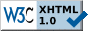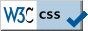Quick Navigation Bar arrays :: dynamic memory allocation :: strings [ toc | forums ] Note: If the document URL does not begin with https://randu.org/tutorials/c/ then you are viewing a copy. Please direct your browser to the correct location for the most recent version.

# Dynamic Memory Allocation

• Now that we have firm grasp on pointers, how can we allocate memory at run-time instead of compile time? ANSI C provides five standard functions that helps you allocate memory on the heap.

## Dynamic Memory Allocation :: sizeof()

• We have already seen this function in the array section. To recap, `sizeof()` returns a `size_t` of the item passed in. So on a typical 32-bit machine, `sizeof(int)` returns 4 bytes. `size_t` is just an unsigned integer constant.
• `sizeof()` is helpful when using `malloc` or `calloc` calls. Note that sizeof() does not always return what you may expect (see below).

## Dynamic Memory Allocation :: malloc(3), calloc(3), bzero(3), memset(3)

• The prototype for `malloc(3)` is:
```  void *malloc(size_t size);
```
`malloc` takes in a `size_t` and returns a void pointer. Why does it return a void pointer? Because it doesn't matter to malloc to what type this memory will be used for.
• Let's see an example of how `malloc` is used:
```  int *ip;

ip = malloc(5 * sizeof(int));
```
Pretty simple. `sizeof(int)` returns the sizeof an integer on the machine, multiply by 5 and malloc that many bytes.
• Wait... we're forgetting something. AH! We didn't check for return values. Here's some modified code:
```  #define INITIAL_ARRAY_SIZE 5
/* ... code ... */
int *ip;

if ((ip = malloc(INITIAL_ARRAY_SIZE * sizeof(int))) == NULL) {
(void)fprintf(stderr, "ERROR: Malloc failed");
(void)exit(EXIT_FAILURE);    /* or return EXIT_FAILURE; */
}
```
Now our program properly prints an error message and exits gracefully if malloc fails.
• `calloc(3)` works like malloc, but initializes the memory to zero if possible. The prototype is:
```  void *calloc(size_t nmemb, size_t size);
```
Refer to Weiss pg. 164 for more information on `calloc`.
• `bzero(3)` fills the first n bytes of the pointer to zero. Prototype:
```  void bzero(void *s, size_t n);
```
If you need to set the value to some other value (or just as a general alternative to bzero), you can use memset:
```  void *memset(void *s, int c, size_t n);
```
where you can specify c as the value to fill for n bytes of pointer s.

## Dynamic Memory Allocation :: realloc(3)

• What if we run out of allocated memory during the run-time of our program and need to give our collection of items more memory?
• Enter `realloc(3)`, it's prototype:
```  void *realloc(void *ptr, size_t size);
```
`realloc` takes in the pointer to the original area of memory to enlarge and how much the total size should be.
• So let's give it a try:
```  ip = realloc(ip, sizeof(ip) + sizeof(int)*5);
```
Now we have some more space through adding the sizeof the complete array and an additional 5 spaces for ints... STOP! This is NOT how you use realloc. Again. The above example is wrong. Why?
• First, sizeof(ip) does not give the size of the allocated space originally allocated by malloc (or a previous realloc). Using sizeof() on a pointer only returns the sizeof the pointer, which is probably not what you intended.
• Also, what happens if the realloc on ip fails? ip gets set to NULL, and the previously allocated memory to ip now has no pointer to it. Now we have allocated memory just floating in the heap without a pointer. This is called a memory leak. This can happen from sloppy realloc's and not using `free` on malloc'd space.
• So what is the correct way? Take this code for example:
```  int *tmp;
if ((tmp = realloc(ip, sizeof(int) * (INITIAL_ARRAY_SIZE + 5))) == NULL) {
/* Possible free on ip? Depends on what you want */
fprintf(stderr, "ERROR: realloc failed");
}
ip = tmp;
```
Now we are creating a temporary pointer to try a realloc. If it fails, then it isn't a big problem as we keep our ip pointer on the original memory space. Also, note that we specified the real size of our original array and now are adding 5 more ints (so 4bytes*(5+5) = 40bytes, on a typical 32-bit machine).

## Dynamic Memory Allocation :: free(3)

• Now that we can `malloc, calloc`, and `realloc` we need to be able to free the memory space if we have no use for it anymore. Like we mentioned above, any memory space that loses its pointer or isn't free'd is a memory leak.
• So what's the prototype for `free(3)`? Here it is:
```  void free(void *ptr);
```
`free` simply takes in a pointer to free. Not challenging at all. Note that `free` can take in NULL, as specified by ANSI.

## Dynamic Memory Allocation :: Multi-dimensional Structures

• It's nice that we can create a ``flat" structure, like an array of 100 doubles. But what if we want to create a 2D array of doubles at runtime? This sounds like a difficult task, but it's actually simple!
• As an example, lets say we are reading in a file of x, y, z coordinates from a file of unknown length. The incorrect method to approach this task is to create an arbitrarily large 2D array with hopefully enough rows or entries. Instead of leaving our data structure to chance, let's just dynamically allocate, and re-allocate on the fly.
• First, let's define a few macros to keep our code looking clean:
```#define oops(s) { perror((s)); exit(EXIT_FAILURE); }
#define MALLOC(s,t) if(((s) = malloc(t)) == NULL) { oops("error: malloc() "); }
#define INCREMENT 10
```
`MALLOC` macro simply takes in the pointer (s) to the memory space to be allocated (t). `oops` is called when `malloc` fails, returning the error code from malloc and exits the program. `INCREMENT` is the default amount of memory to allocate when we run out of allocated space.
• On to the dynamic memory allocation!
```  double **xyz;
int i;

MALLOC(xyz, sizeof(double *) * INCREMENT);
for (i = 0; i < INCREMENT; i++) {
MALLOC(xyz[i], sizeof(double) * 3);
}
```
What's going on here? Our double pointer, `xyz` is our actual storage 2D array. We must use a double pointer, because we are pointing to multiple pointers of doubles! If this sounds confusing, think of it this way. Instead of each array entry having a real double entry, each array position contains a pointer to another array of doubles! Therefore, we have our desired 2D array structure.
• The first `MALLOC` call instructs malloc to create 10 double pointers in the xyz array. So each of these 10 array positions now has an unitializied pointer to data of type pointer to a double. The `for` loop goes through each array position and creates a new array at each position to three doubles, because we want to read in x, y, z coordinates for each entry. The total space we just allocated is 10 spaces of 3 doubles each. So we've just allocated 30 double spaces.
• What if we run out of space? How do we reallocate?
```  double **tmp;
int current_size, n;

/* clip ... other code */

if (current_size >= n) {
if ((tmp = realloc(xyz, sizeof(double *) * (n + INCREMENT)) == NULL) {
oops("realloc() error! ");
}
for (i = n; i < n + INCREMENT; i++) {
MALLOC(tmp[i], sizeof(double) * 3);
}
n += INCREMENT;
xyz = tmp;
}
```
• What's going on here? Suppose our file of x, y, z coordinates is longer than 10 lines. On the 11th line, we'll invoke the `realloc()`. `n` is the current number of rows allocated. `current_size` indicates the number of rows we are working on (in our case, the expression would be 10 >= 10). We instruct `realloc` to reallocate space for xyz of (double *) type, or double pointers of the current size (n) plus the `INCREMENT`. This will give us 10 additional entries. Remember NEVER reallocate to the same pointer!!
• If `realloc()` succeeds, then we need to allocate space for the double array of size 3 to hold the x, y, z coordinates in the new xyz realloc'd array. Note the for loop, where we start and end. Then we cleanup by providing our new max array size allocated (n) and setting the xyz double pointer to the newly realloc'd and malloc'd space, `tmp`.
• Not as difficult as you might have imagined it to be, right? What if we're done with our array? We should free it!
```  for (i = 0; i < n; i++) {
free(xyz[i]);
}
free(xyz);
```
The above code free's each entry in the xyz array (the actual double pointers to real data) and then we free the pointer to a pointer reference. The statements cannot be reversed, because you'll lose the pointer reference to each 3-entry double array!

# Dynamic Memory Allocation Review

• You have powerful tools you can use when allocating memory dynamically: `sizeof, malloc, calloc, realloc`, and `free`.
• Take precautions when using the actual memory allocation functions for memory leaks, especially with realloc. Remember, always check for NULL with malloc! Your programs will thank you for it.

Notice: Please do not replicate or copy these pages and host them elsewhere. This is to ensure that the latest version can always be found here.

Disclaimer: The document author has published these pages with the hope that it may be useful to others. However, the document author does not guarantee that all information contained on these webpages are correct or accurate. There is no warranty, expressed or implied, of merchantability or fitness for any purpose. The author does not assume any liability or responsibility for the use of the information contained on these webpages.

If you see an error, please send an email to the address below indicating the error. Your feedback is greatly appreciated and will help to continually improve these pages.

© 1999-2023 Alfred Park (fred [ANTISPAM-REMOVE-THIS] AT randu.org)